# Expanding Binomial Products

Welcome to the first mathematical post in The Mathenæum Blog. In this post, I have chosen to discuss expanding binomial products, because in many ways binomial products act as a bridge between elementary algebraic work, and more advanced applications in equation solving and curve plotting. But also, and this will certainly not surprise my former students, because it leads nicely to a couple of interesting bamboozlings!

A binomial is just a sum or difference of two terms: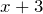,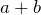, and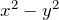are all examples. Think bi-nomial as in bi-cycle, bi-centenary, bi-lateral. Binomials are important because of the role they often play in the reduction of more complicated expressions to forms that are more easily understood and analysed. Such reductions are central to factoring quadratics and higher order poly-nomials, plotting parabolas and more general curve sketching, and solving nonlinear equations. Binomials also play a very important role in Probability Theory, specifically when calculating probabilities for sequences of binary choices (notice bi-nary) such as flipping a coin (see Binomial Bomber).

Stepping back a bit, recall these three important laws of arithmetic:

1. The commutative law tells us that when we add or multiply two numbers, it doesn’t matter which one comes first: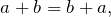Remember this because to commute means to go back and forth.

2. The associative law tells us that when we add or multiply three numbers, it doesn’t matter if we add the first two then the third, or the last two then the first: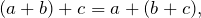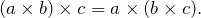You can remember this law since to associate means to go together.

3. The distributive law tells us how to expand an expression with brackets:Because of the commutative law, this is just the same asand sometimes it is easier to use in this form. You can remember the name of this law since to distribute is to spread out.

Now suppose we have a binomial product to expand, such as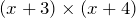but we usually leave the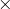out and simply write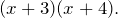This doesn’t immediately look very much like any of those laws, but the distributive law is closest since it tells us how to “distribute” over a term in brackets. So let’s just try using it exactly as we would for a simpler expression, i.e. multiplying all of the first bracketed term by each part of the binomial in the second set of brackets individually, and see what we get. This gives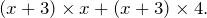Now we have two separate terms, each of which can be easily expanded using the distributive law again to get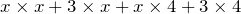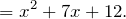And that is how you expand a binomial expression — just like expanding a single set of brackets, but twice. Also, notice how the numbers we started with were 3 and 4, but we ended with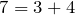and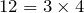. That’s a big clue about how binomial products mix terms together, and is crucial to applying the reverse process of factorisation. Mathematics is the art of discovering and explaining patterns, so always be on the look out for things like this. They are the key to mathematical understanding.

## Three Binomial Identities

An identity in mathematics is an equality that is always true no matter what value is substituted for any unknowns it includes. There are three important binomial product identities — you can verify each by expanding as we have just done.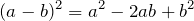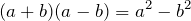Recognising these forms within an algebraic expression can be very useful when factorising or otherwise attempting to understand or describe its properties.

## What have we learned?

We have seen how to expand a binomial product, but that is not all we have learned. Indeed, it is not even the most important thing we have learned. The most important thing this process has illustrated is that often something that appears new is actually just the same as what you already know. All we did was apply the distributive law to the product, just as we would to any simpler product. It was exactly the same process, just applied more than once. There was no new mathematics at all, and we did not need to learn or memorise any new rules! Knowing how to expand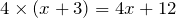meant we already knew everything we needed to calculate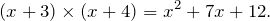Don’t be surprised by this, but be confident in your mathematical knowledge and experience, and be prepared to attack problems that look new. Every bit of progress you make is satisfying and enjoyable, teaches you more about maths than memorising rules and examples, and sticks in your mind better as well. It’s a win all round!

## Prepare to be bamboozled!

Let’s look at some fun false proofs that rely on binomial identities to prove ridiculous statements. See if you can spot the errors.

#### Bamboozler #1: Proof that 1 = 2

Suppose we have pronumerals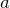and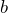such that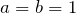. Then clearlyMultiply both sides byto getSubtract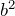from both sides to get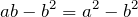Now the left hand side has a common factor of, and the right hand side is in the form of the third of our identities, so we can write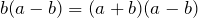and dividing out the common factor of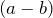givesSince bothandequal 1, we have shown that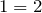### Click to show/hide explanation

Since, dividing byis dividing by zero and thus invalid. Think about it; if dividing by zero was allowed, you could prove any number equals any other number by simply noting that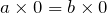, then cancelling the zeroes to get.

#### Bamboozler #2: Proof that 2 = 4

Can you find the flaw in the following reasoning?

It is obviously true that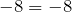and therefore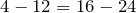We can rewrite this asand adding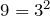to both sides gives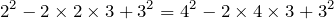Hmmmm, anything familiar about those expressions? They look a little like our second binomial identity don’t you think? They certainly do — just set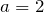for the left hand side,for the right hand side,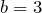for both, and we can rewrite our equation in the simplified form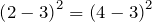Taking the square root of both sides leaves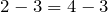and finally, by addingto both sides, we have shown that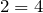### Click to show/hide explanation

The error in this case comes from taking positive square roots only in the second last step. Any positive number has both a positive and a negative square root, since a negative times a negative is a positive. For example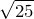is both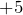and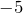, but just because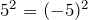, we obviously can’t conclude thatThe same is true for our “proof”, just made a little more obscure by the binomials.Mr Wessen

Sometime teacher, sometime programmer, full time mathematician.